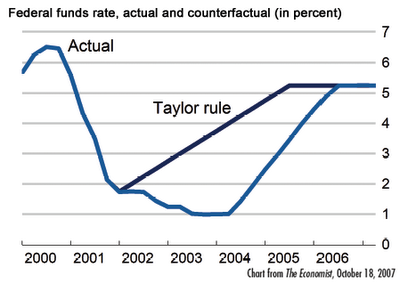## Wednesday, May 13, 2009

### Taylor Rule and Interest Rates

The Taylor rule is a formula for setting interest rates depending on changes in the inflation rate and economic growth.

A simplified formula is: r = p + 0.5y + 0.5 (p - 2) + 2 (after Tobin, 1998)
• r = the short term interest rate in percentage terms per annum.
• p = the rate of inflation over the previous four quarters.
• y = the difference between real GDP from potential output.
• This assumes that target inflation is 2% and equilibrium real interest rate is 2%

Example of Taylor Rule:
• If inflation were to rise by 1%, the Taylor response would be to raise the interest rate by about 1.5%
• If GDP falls by 1% relative long run trend rate, then the Taylor response is to cut the interest rate by about 0.5%
• Basically, higher growth and inflationary pressures require higher interest rates to reduce economic activity. Lower growth and a fall in inflation require lower interest rates to boost spending.
Using this formula, it is clear that in the period 2002-04, the US Federal Reserve kept interest rates too low. These low interest rate were a key factor (though by no means the only cause) of a boom in credit and a boom in house prices.

### Interest Rates Too Low in US Using Taylor RuleThe interesting thing is what the Taylor rule says about current interest rates. Since GDP in US has collapsed by - 4% (when growth trend is about 2%) it means that GDP is much lower than potential. Paul Krugman estimated that using the Taylor rule, the US should give a nominal interest rate of -7%. This indicates why the Federal Reserve are having to resort to quantitative easing. They can't cut interest rates below 0%, so they need to resort to unorthodox measures to boost the economy.

### Taylor Rule and Suggested Interest Rate for US